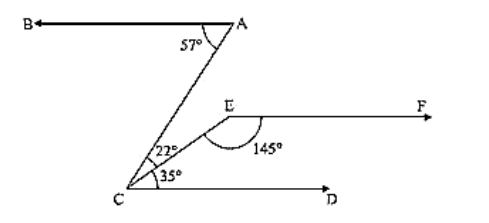# In the below fig, show that AB ∥ EF

Question:

In the below fig, show that AB ∥ EFSolution:

Produce EF to intersect AC at K.

Now, ∠DCE + ∠CEF = 35 + 145 = 180°

Therefore, EF ∥ CD (Since Sum of Co-interior angles is 180) ... (1)

Now, ∠BAC = ∠ACD = 57°

⟹ BA ∥ EF             [Alternative angles are equal] ... (2)

From (1) and (2)

AB ∥ EF [Since, Lines parallel to the same line are parallel to each other]

Hence proved.